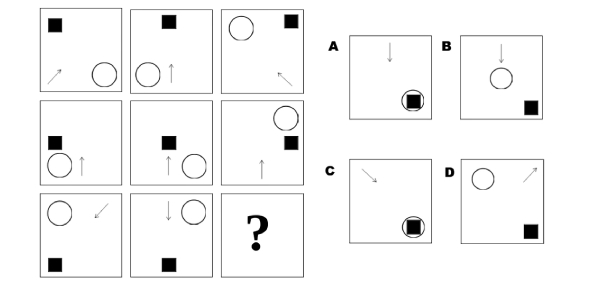# Maths Logical Reasoning Trivia Quiz

10 Questions | Attempts: 1018
ShareSettingsAre you familiar with logical reasoning and think you can pass this Maths trivia quiz? Check it out now. Logical reasoning is one of the essential skills of practical thinking. Logic can also be explained as reasoning conducted according to rigorous principles of validity. A valid argument has a specific relation of logical support between the argument's assumptions and its conclusion. If you need to learn more about logical reasoning, this quiz can help to make your learning better.

• 1.
If I had 50c and I bought a pencil for 25c what change will I get?
• A.

30c

• B.

20c

• C.

25c

• D.

35c

• 2.
If today is Sunday, what day will it be in 8 days?
• A.

Sunday

• B.

Friday

• C.

Monday

• D.

Thursday

• 3.
If I cut a pizza into 1/4 how much slices will there be?
• A.

6

• B.

4

• C.

2

• D.

7

• 4.
If the school was over at 3oclock and it was half 4 when tom got home how long did it take for tom to get home?
• A.

1 hour

• B.

1 hour and a half hours

• C.

2 hours

• D.

2 and a half hours

• 5.
Tom had 30 apples and he gave Jack half of it, how much did Jack get?
• A.

10 apples

• B.

15 apples

• C.

20 apples

• D.

25 apples

• 6.
The teacher let the kids play with the iPods for 20 minutes, then it was home time. What time was it when the teacher gave the iPods out?
• A.

20 past 2

• B.

Half 2

• C.

2 o'clock

• D.

5 o'clock

• 7.
Tom went to sleep at 10 o'clock and woke up at 9 o'clock. How long did he sleep?
• A.

15 hours

• B.

11 hours

• C.

7 hours

• D.

12 hours

• 8.
I had £2 and bought a bag of oranges for £1:30, what change did I get?
• A.

55c

• B.

30c

• C.

70c

• D.

40c

• 9.
What number should come next in this series: 22, 21, 23, 22, 24, 23, ...?
• A.

23

• B.

24

• C.

25

• D.

26

• 10.
If 13 x 12 = 651 & 41 x 23 = 448, then, 24 x 22 =?
• A.

524

• B.

534

• C.

924

• D.

1024

## Related TopicsBack to top
×

Wait!
Here's an interesting quiz for you.